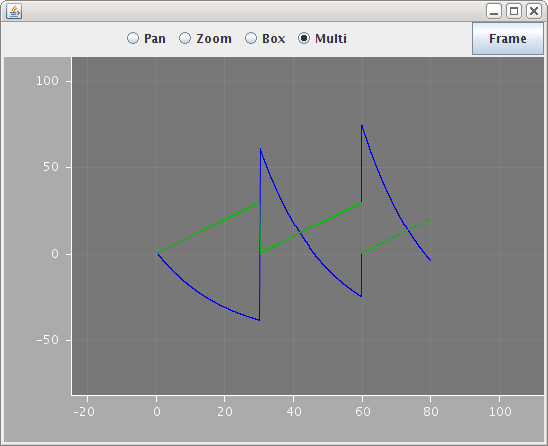# User defined types for networks and populations

This example shows how the standard component type structures can be used to declare components for simple networks. The following three definitions allow networks to be constructed containing fixed size populations of a particular component type.

<ComponentType name="Network">
<Children name="populations" type="Population" />
<Children name="connectivities" type="EventConnectivity" />
</ComponentType>
<ComponentType name="Population">
<ComponentRef name="component" type="Component" />
<Parameter name="size" dimension="none" />
</ComponentType>
<ComponentType name="EventConnectivity">
<Child name="Connections" type="ConnectionPattern" />
</ComponentType>

The harder part is to provide elements in the Dynamics blocks to express what should be done with components based on these types. The Network element doesn't pose any problems because the default behavior on instantiation will do the right thing: it will instantiate each of the child populations and EventConnectivity elements.

But the population element needs to say that its instantiation involves making 'size' instances of the component referred to by the 'component' reference, where 'size' is the value supplied for the size parameter in a component specification. This can be done by including a Build element inside the Dynamics block:

<ComponentType name="Population">
<ComponentRef name="component" type="Component" />
<Parameter name="size" dimension="none" />
<Dynamics>
<Build>
<MultiInstantiate number="size" component="component" />
</Build>
</Dynamics>
</ComponentType>

The MultiInstantiate specification says that there should be 'size' instances of the component referred to in the 'component' parameter created when the model is built. This overrides the default behavior. [TODO: what is the Build element content corresponding to the default behavior?].

This serves to create some rather simple populations. More complex specifications, such as putting one instance at each point of a grid satisfying a particular constraint could be handled via first declaring elements to form the grid, and then using selectors that pick the points in the population element to actually put the cells at [its not clear to me how much more would be required to make this work, other than implementing proper xpath-like selectors].

The following three types define a general connectivity structure with an abstract ConnectionPattern type, and a specific instance for All-All connectivity.

<ComponentType name="EventConnectivity">
<Child name="Connections" type="ConnectionPattern" />
</ComponentType>
<ComponentType name="ConnectionPattern">
</ComponentType>
<ComponentType name="AllAll" extends="ConnectionPattern">
<Dynamics>
<Build>
<ForEach instances="../source" as="a">
<ForEach instances="../target" as="b">
<EventConnection from="a" to="b" />
</ForEach>
</ForEach>
</Build>
</Dynamics>
</ComponentType>

The Build element in the AllAll pattern uses a new ForEach construct and the EventConnectin element from before. The ForEach element operates selects each instance matching its 'instances' attribute, and applies the enclosing directives, much in the same way as for-each in XSL. The proof of concept interpreter also has Choose, When and Otherwise elements that operate much like their XSL equivalents, although these are not used in this example.

With these definitions in place, a network simulation can be defined with the following:

<Network id="net1">
<Population id="p1" component="gen1" size="2" />
<Population id="p3" component="iaf3cpt" size="3" />
<EventConnectivity id="p1-p3" source="p1" target="p3">
<Connections type="AllAll" />
</EventConnectivity>
</Network>

<Simulation id="sim1" length="80ms" step="0.05ms" target="net1">
<Display timeScale="1ms">
<Line id="gen_v" quantity="p3/v" scale="1mV" color="#0000f0" />
<Line id="gen_tsince" quantity="p1/tsince" scale="1ms" color="#00c000" />
</Display>
</Simulation>

The output when the model is run is shown below, followed by the full listing.example7.xml (xml)
<Lems>
<Target component="simset" />
<Include file="ex2dims.xml" />
<Include file="spikegenerators.xml" />
<Include file="misciaf.xml" />

<Component id="gen1" type="spikeGenerator" period="30ms" />

<Component id="gen2" type="spikeGenerator2" period="32ms" />

<Component id="iaf3cpt" type="iaf3" leakReversal="-50mV" deltaV="50mV" threshold="-30mV" leakConductance="50pS" refractoryPeriod="4ms" capacitance="1pF" />
<ComponentType name="Network">
<Children name="populations" type="Population" />
<Children name="connectivities" type="EventConnectivity" />
</ComponentType>
<ComponentType name="Population">
<ComponentReference name="component" type="Component" />
<Parameter name="size" dimension="none" />
<Structure>
<MultiInstantiate number="size" component="component" />
</Structure>
</ComponentType>
<ComponentType name="EventConnectivity">
<Child name="Connections" type="ConnectionPattern" />
</ComponentType>
<ComponentType name="ConnectionPattern" />
<ComponentType name="AllAll" extends="ConnectionPattern">
<Structure>
<ForEach instances="../source" as="a">
<ForEach instances="../target" as="b">
<EventConnection from="a" to="b" />
</ForEach>
</ForEach>
</Structure>
</ComponentType>

<Network id="net1">
<Population id="p1" component="gen1" size="2" />
<Population id="p3" component="iaf3cpt" size="3" />
<EventConnectivity id="p1-p3" source="p1" target="p3">
<Connections type="AllAll" />
</EventConnectivity>
</Network>
<Include file="MultiRunSimulation.xml" />
<SimulationSet id="simset">
<Display id="disp1" title="Example 7: User defined types for networks and populations" xmin="0" xmax="80" ymin="-80" ymax="50" />

<Simulation id="sim1" length="80ms" step="0.05ms" target="net1" display="disp1">
<DisplayList timeScale="1ms" title="">
<Line id="gen_v" quantity="p3/v" scale="1mV" timeScale="1ms" color="#0000f0" />
<Line id="gen_tsince" quantity="p1/tsince" scale="1ms" timeScale="1ms" color="#00c000" />
</DisplayList>
</Simulation>
</SimulationSet>
</Lems>